Q-Chem 5.1 User’s Manual

# 5.2 Kohn-Sham Density Functional Theory

The density functional theory by Hohenberg, Kohn, and Sham stems from earlier work by Dirac, who showed that the exchange energy of a uniform electron gas can be computed exactly from the charge density along. However, while this traditional density functional approach, nowadays called “orbital-free” DFT, makes a direct connection to the density alone, in practice it is constitutes a direct approach where the necessary equations contain only the electron density, difficult to obtain decent approximations for the kinetic energy functional. Kohn and Sham sidestepped this difficulty via an indirect approach in which the kinetic energy is computed exactly for a noninteracting reference system, namely, the Kohn-Sham determinant. It is the Kohn-Sham approach that first made DFT into a practical tool for calculations.

Within the Kohn-Sham formalism, the ground state electronic energy,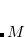, can be written as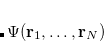(5.1)

where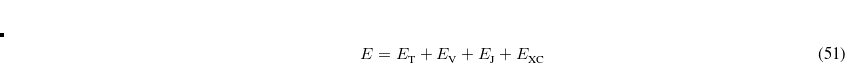is the kinetic energy,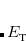is the electron–nuclear interaction energy,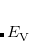is the Coulomb self-interaction of the electron density,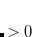and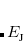is the exchange-correlation energy. Adopting an unrestricted format, the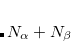and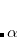total electron densities can be written as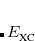(5.2)

where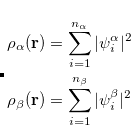and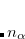are the number of alpha and beta electron respectively, and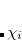are the Kohn-Sham orbitals. Thus, the total electron density is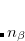(5.5)

Within a finite basis set, the density is represented by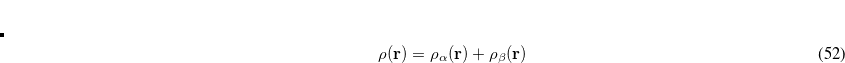(5.6)

where theare the elements of the one-electron density matrix; see Eq. eq:P(mu,nu) in the discussion of Hartree-Fock theory. The various energy components in Eq. eq:DFT_energy_components can now be written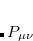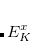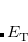(5.7)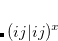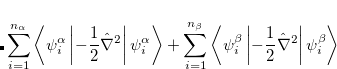(5.8)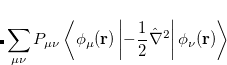(5.9)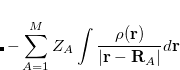(5.10)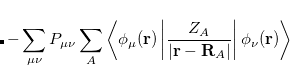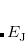(5.11)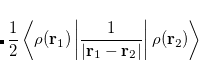(5.12)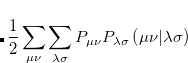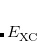(5.13)

Minimizingwith respect to the unknown Kohn-Sham orbital coefficients yields a set of matrix equations exactly analogous to Pople-Nesbet equations of the UHF case, Eq. eq:Pople-Nesbet, but with modified Fock matrix elements [cf. Eq. eq:F(mu,nu)]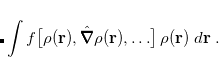(5.14)

Here,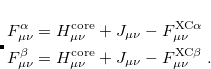and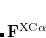are the exchange-correlation parts of the Fock matrices and depend on the exchange-correlation functional used. UHF theory is recovered as a special case simply by taking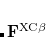, and similarly for. Thus, the density and energy are obtained in a manner analogous to that for the HF method. Initial guesses are made for the MO coefficients and an iterative process is applied until self-consistency is achieved.